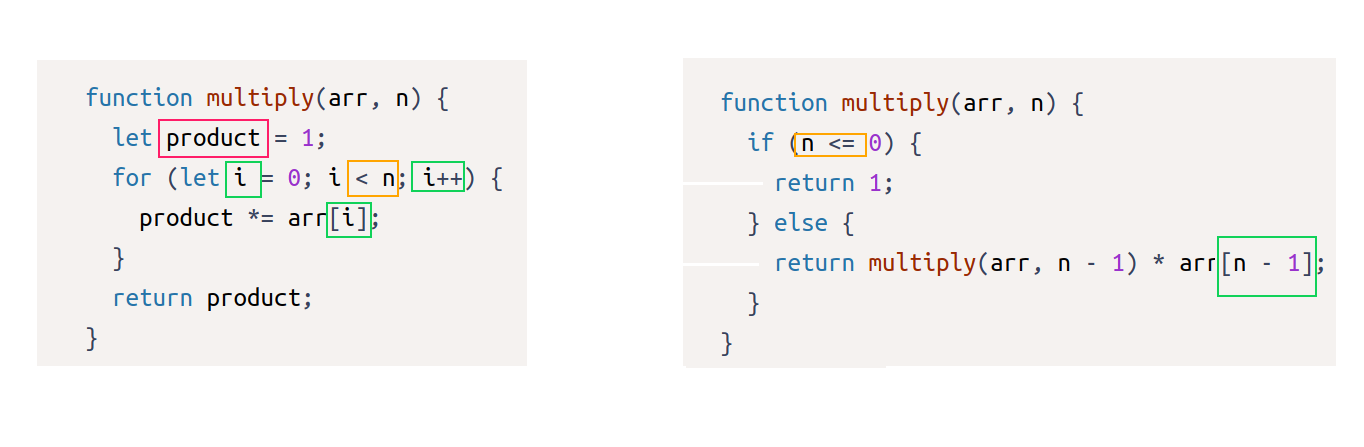# Basic JavaScript - Replace Loops using Recursion

Tell us what’s happening:
Describe your issue in detail here.

Hello everyone, pretty excited and gratefull for all of the job done by you guys, really enjoying it!!!

Don´t know where my questions goes/comes to/from, but I cannot understand why is that `multiply(arr, n) == multiply(arr, n - 1) * arr[n - 1]`.:

``````function multiply(arr, n) {
let product = 1;
for (let i = 0; i < n; i++) {
product *= arr[i];
}
return product;
}
``````

And says: " notice that `multiply(arr, n) == multiply(arr, n - 1) * arr[n - 1]`. That means you can rewrite `multiply` in terms of itself and never need to use a loop."—>Where do you get this??? could someone please explain???

Ty so much for the time u take to answer``````function sum(arr, n) {
// Only change code below this line

// Only change code above this line
}
``````

User Agent is: `Mozilla/5.0 (Windows NT 10.0; Win64; x64; rv:108.0) Gecko/20100101 Firefox/108.0`

Challenge: Basic JavaScript - Replace Loops using Recursion

I believe your question is somewhat vague.
I’m going to guess that your real question is: where is multiply calling itself in the code?
It is calling itself in the -re-written- code that you did not quote above.
Here it is from the exercise

``````  function multiply(arr, n) {
if (n <= 0) {
return 1;
} else {
return multiply(arr, n - 1) * arr[n - 1];
}
}
``````

ps. if I guessed your question’s meaning incorrectly, please rephrase it so we can answer it better.

1 Like

multiply (for loop,left) vs multiply (recursion, right):what are the differences between the 2 versions of `multiply`?

Note:
I have made a video explaining (step by step) this lesson freeCodecamp: "Replace Loops Using Recursion", Solution. - YouTube .

1 Like